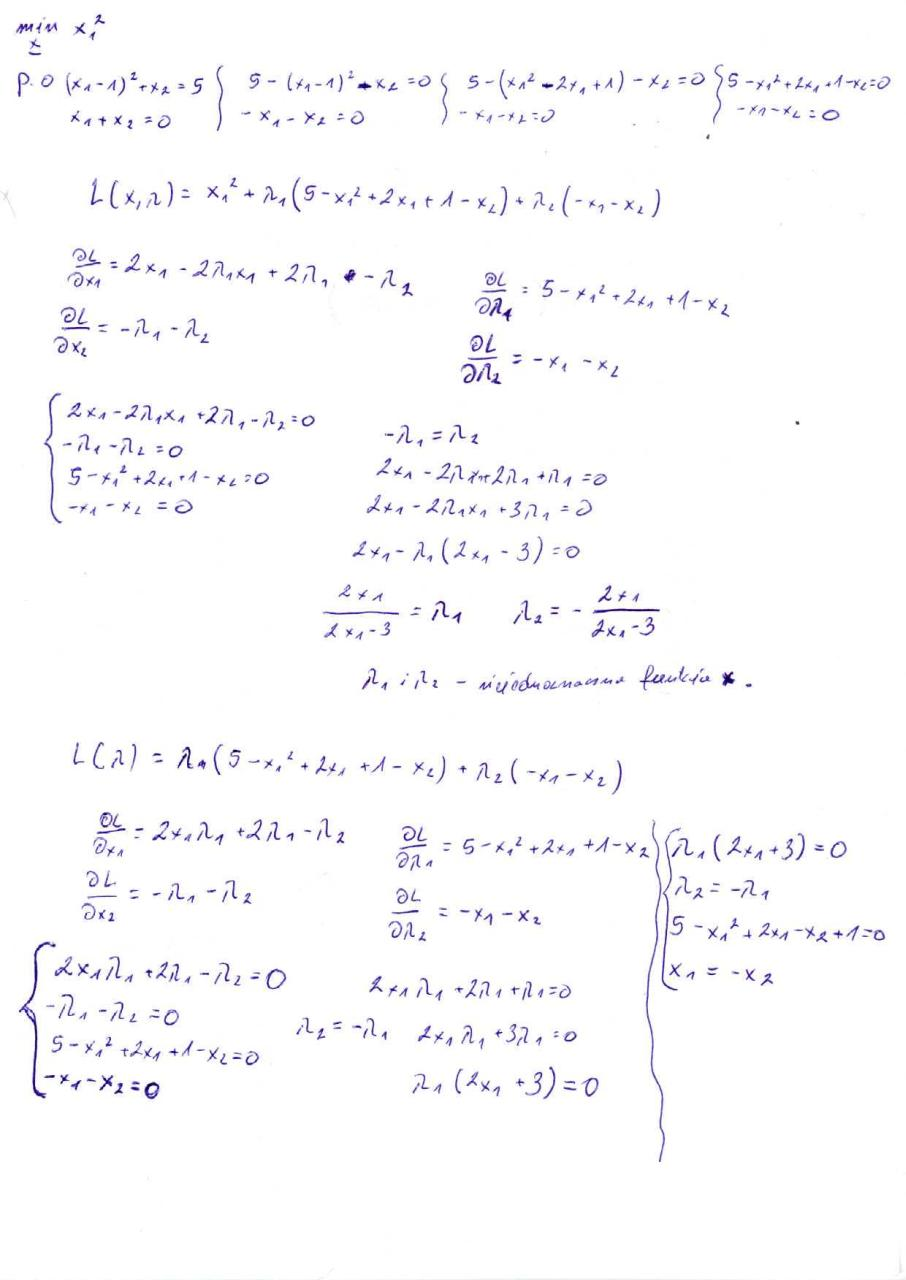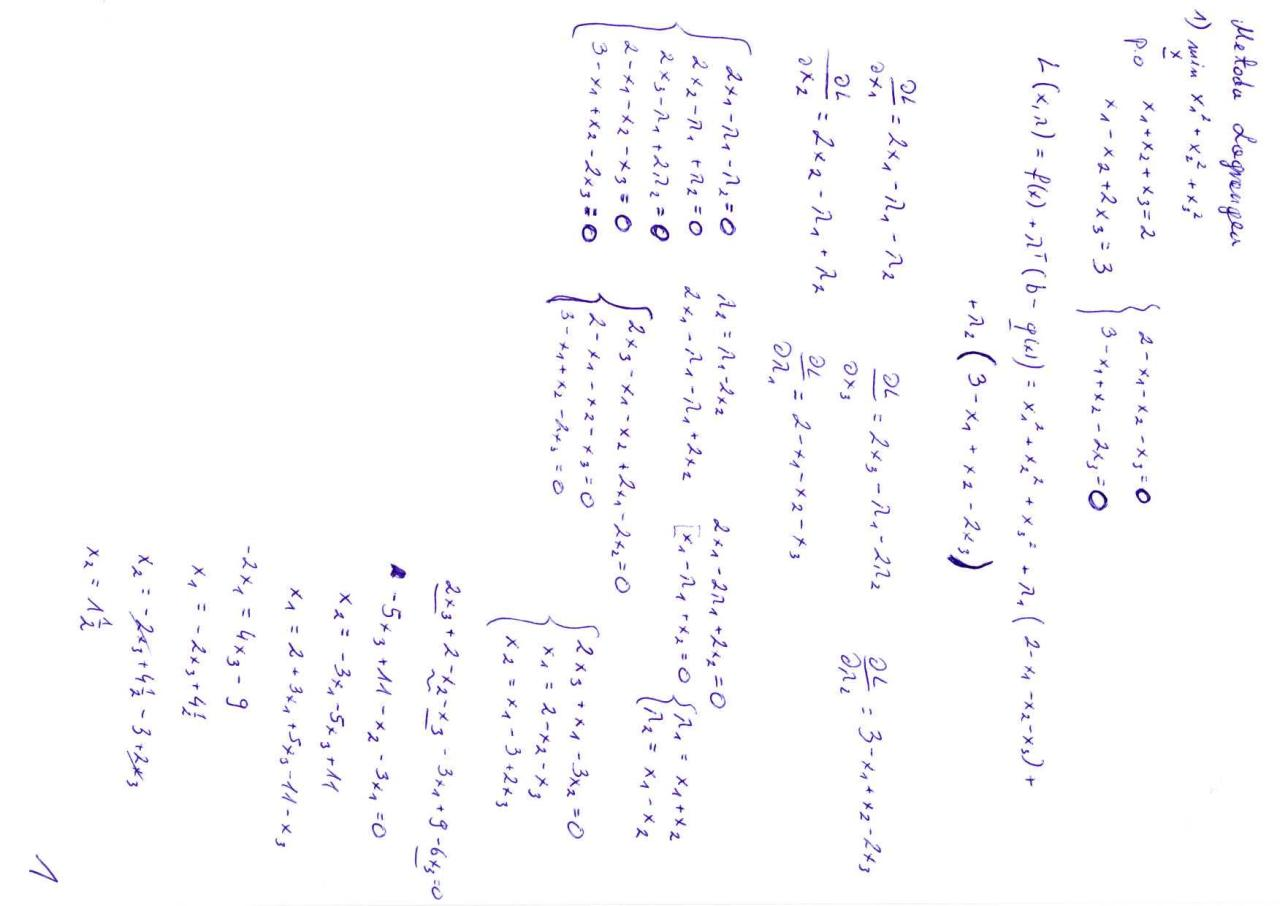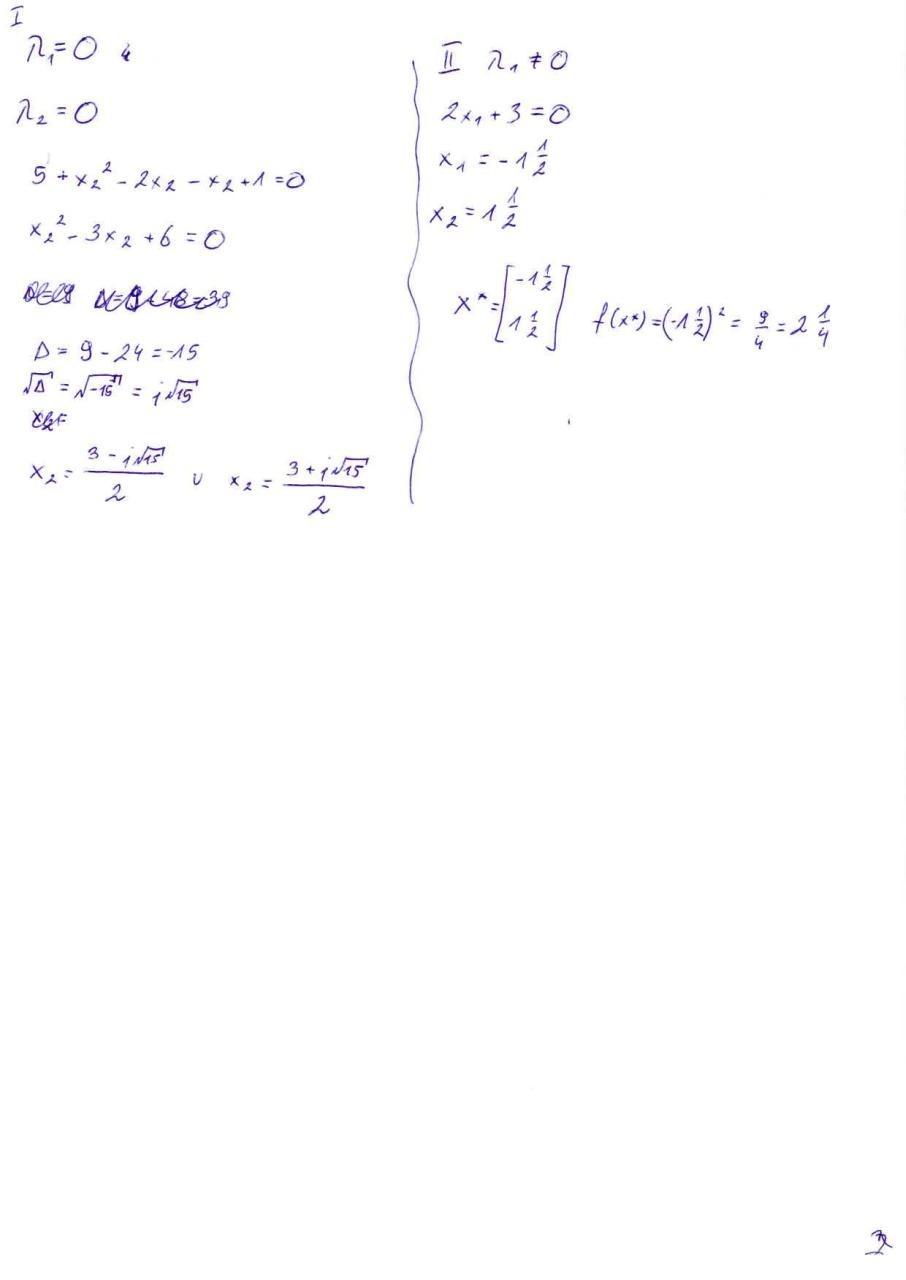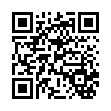# Lag1 .pdf

### File information

Original filename: Lag1.pdf

This PDF 1.2 document has been generated by Sprint / Unknown, and has been sent on pdf-archive.com on 04/06/2011 at 12:28, from IP address 89.228.x.x. The current document download page has been viewed 858 times.
File size: 450 KB (3 pages).
Privacy: public file

Lag1.pdf (PDF, 450 KB)

### Document preview

.o t-

S -*/,z_

Ł ( X, /l ) = */

S-

-x J

-o
L

~

-&amp; (A*&lt; -3) -'O
*
l

ii

,

/U = " •

-3

- M

/ l * J / l i -A
A*r -71 -r
5-X/4*

**

Vn
r

1

\

^

X

U

x
^

~*

-.,

~-

.0

A

_-&gt;

K*

v
0" O
O
r\ ^&gt;
£ O
£

**~*
Ui
1
Ifc.

A

+

X
K
J
\
S

f-

u-

ti

0

x

X

?^4

^-^

K ^

i

x *

M I

r" *

»

x

y

^

'

*

^ ^.
"
X^
:
^ •*
p,

A.

i

xl

v!

&gt;

+

&gt;
"
^

i

,

^

A^

,
^

&gt;o

&gt;

^

r^A

i CA

v.

1,

v

n

U

K)

lrx

\,

-^
'
&gt;0^

1

c

V
K.-*

u

X

^
,1

"^J

bi

i
X,
-i
+
~K

K

XJ
~\
N
' ,
Cr/

x,
'

x
v.
"

^

o „
o

li

l

^

K
U.

x
+

^

X

i

o

X

X

^

X
N

Ko

I/

Ii

N

V Ł\
VS1

JX

-f

x.

N

V.
iSJi

1

X

J-

'

K

Vi

1

^\

£
U,

&lt;-.

k

X

X,

*-

j

1

l
X

i

4
x
v,

^

,
xX
V

-t
X*
HX

^
4
K&gt;

f—-„

•^

O

*

C

'-*

\i

V
V

x
X.

ł

T

^ S? o , ^

*

^s
K^

*

"^

X

'
'
.
' 4x^
*
uX
xj "^&gt;

4

^

X.

li
-i_

x

^x

*

^ v

?0

~

*

"*

f•

X,

l|

0

X

"

^&gt;

X,r-

y#### HTML Code

Copy the following HTML code to share your document on a Website or Blog

#### QR Code### Related keywords